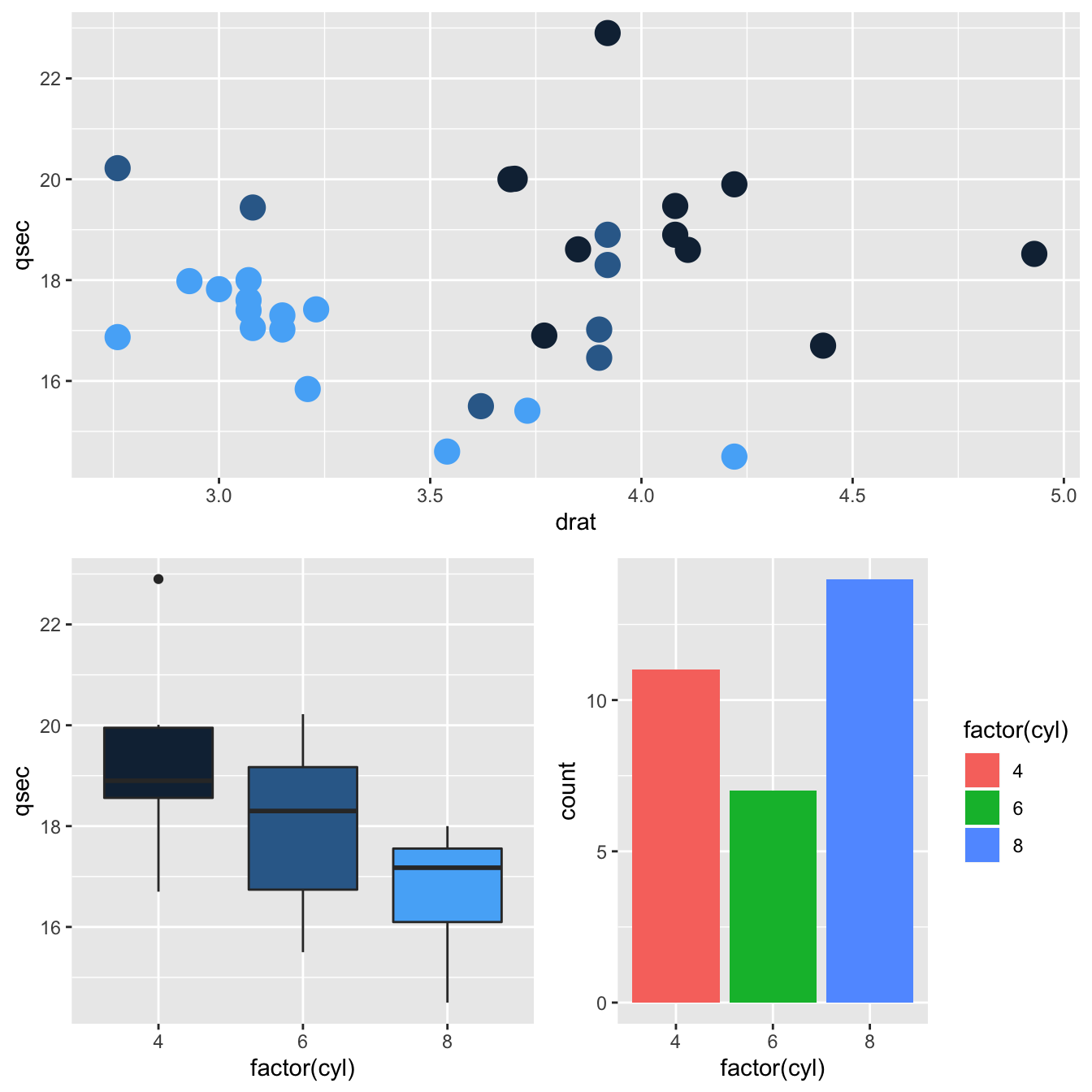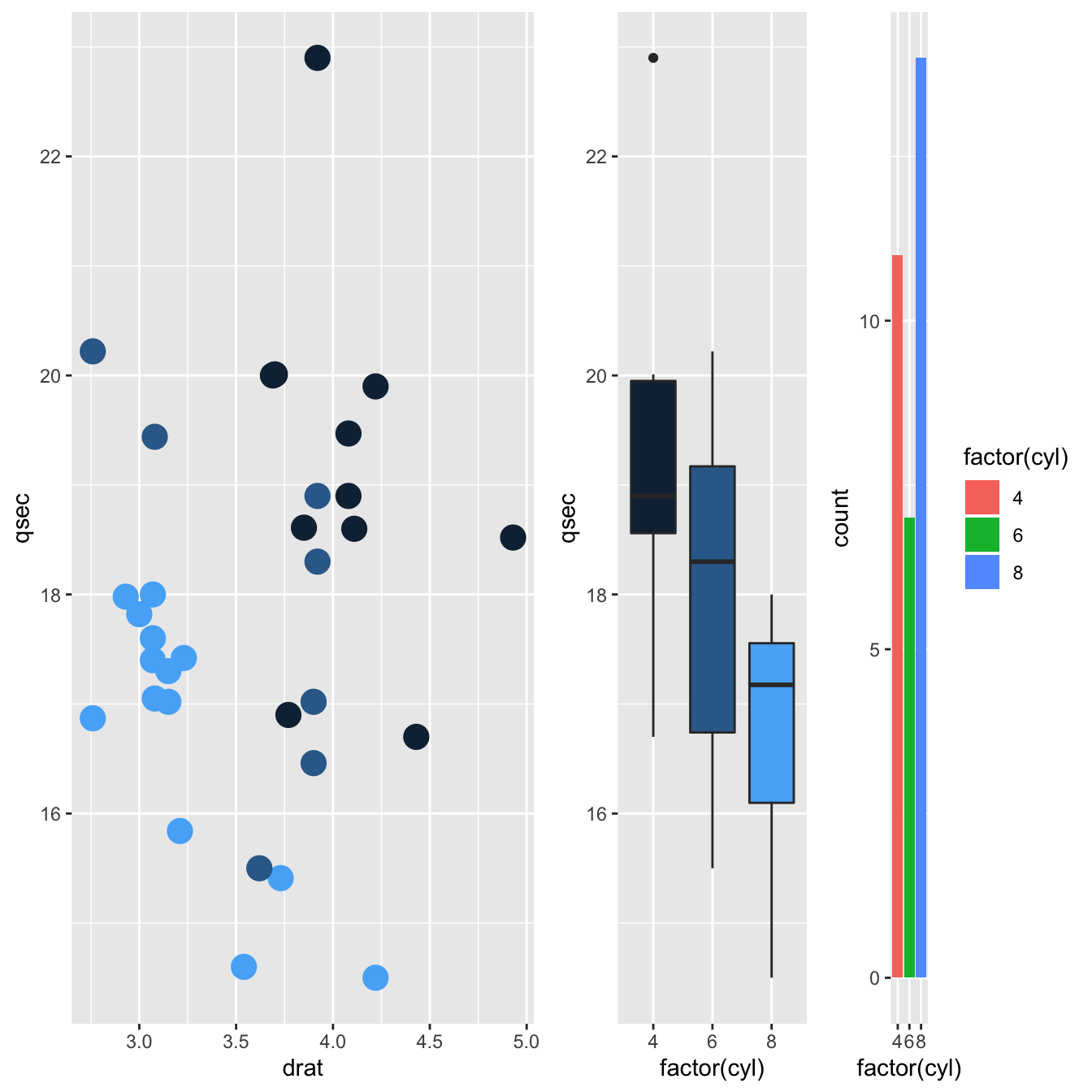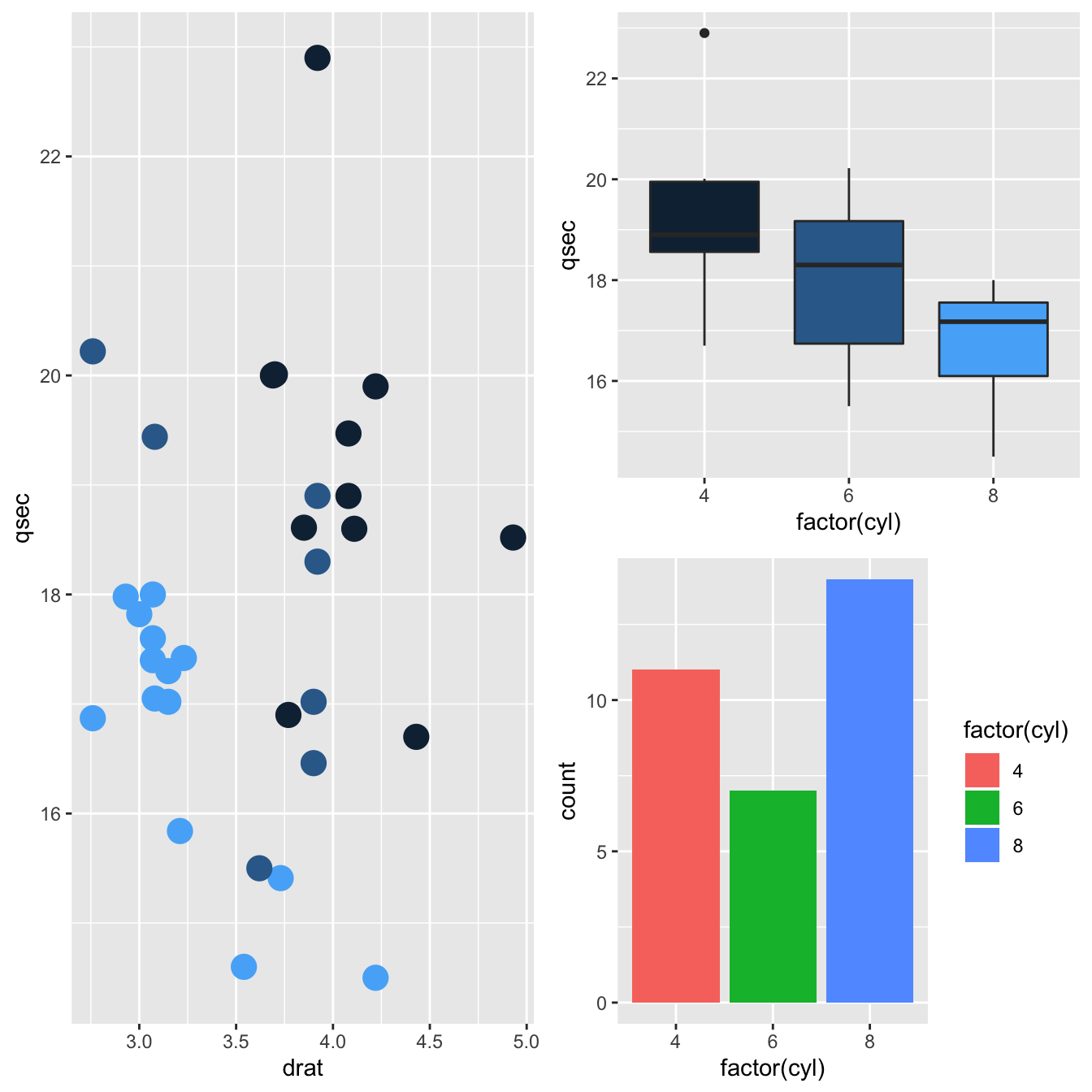# Multiple ggplot2 charts on a single page

This post shows how to use the `gridExtra` library to combine several `ggplot2` charts on the same figure. Several examples are provided, illustrating several ways to split the graphing window.

Mixing multiple graphs on the same page is a common practice. It allows to summarize a lot of information on the same figure, and is for instance widely used for scientific publication.

The gridExtra package makes it a breeze. It offers the `grid.arrange()` function that does exactly that. Its `nrow` argument allows to specify how to arrange the layout.

For more complex layout, the `arrangeGrob()` functions allows to do some nesting. Here are 4 examples to illustrate how `gridExtra` works:``````# libraries
library(ggplot2)
library(gridExtra)

# Make 3 simple graphics:
g1 <- ggplot(mtcars, aes(x=qsec)) + geom_density(fill="slateblue")
g2 <- ggplot(mtcars, aes(x=drat, y=qsec, color=cyl)) + geom_point(size=5) + theme(legend.position="none")
g3 <- ggplot(mtcars, aes(x=factor(cyl), y=qsec, fill=cyl)) + geom_boxplot() + theme(legend.position="none")
g4 <- ggplot(mtcars , aes(x=factor(cyl), fill=factor(cyl))) +  geom_bar()

# Plots
grid.arrange(g2, arrangeGrob(g3, g4, ncol=2), nrow = 2)``````
``grid.arrange(g1, g2, g3, nrow = 3)``
``grid.arrange(g2, arrangeGrob(g3, g4, ncol=2), nrow = 1)``
``grid.arrange(g2, arrangeGrob(g3, g4, nrow=2), nrow = 1)``

Related chart types

## Contact

This document is a work by Yan Holtz. Any feedback is highly encouraged. You can fill an issue on Github, drop me a message on Twitter, or send an email pasting yan.holtz.data with gmail.com.